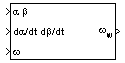# Wind Angular Rates

Calculate wind angular rates from body angular rates, angle of attack, sideslip angle, rate of change of angle of attack, and rate of change of sideslip

• Library:
• Aerospace Blockset / Flight Parameters

•## Description

The Wind Angular Rates block supports the equations of motion in wind-fixed frame models by calculating the wind-fixed angular rates (pw, qw, rw). For more information on the equation used for the calculation, see Algorithms.

## Ports

### Input

expand all

Angle of attack and sideslip, specified as a 2-by-1 vector, in radians.

Data Types: `double`

Rate of change of the angle of attack and rate of change of the sideslip, specified as a 2-by-1 vector, in radians per second.

Data Types: `double`

Body angular rates, specified as a three-element vector, in radians per second.

Data Types: `double`

### Output

expand all

Wind angular rates, returned as a three-element vector, in radians per second.

Data Types: `double`

## Algorithms

The body-fixed angular rates (pb, qb, rb), angle of attack $\left(\alpha \right)$, sideslip angle (β), rate of change of angle of attack $\left(\stackrel{˙}{\alpha }\right)$, and rate of change of sideslip $\left(\stackrel{˙}{\beta }\right)$ are related to the wind-fixed angular rates as illustrated in the following equation:

`$\left[\begin{array}{c}{p}_{w}\\ {q}_{w}\\ {r}_{w}\end{array}\right]=\left[\begin{array}{ccc}\mathrm{cos}\alpha \mathrm{cos}\beta & \mathrm{sin}\beta & \mathrm{sin}\alpha \mathrm{cos}\beta \\ -\mathrm{cos}\alpha \mathrm{sin}\beta & \mathrm{cos}\beta & -\mathrm{sin}\alpha \mathrm{sin}\beta \\ -\mathrm{sin}\alpha & 0& \mathrm{cos}\alpha \end{array}\right]\left[\begin{array}{c}{p}_{b}-\stackrel{˙}{\beta }\mathrm{sin}\alpha \\ {q}_{b}-\stackrel{˙}{\alpha }\\ {r}_{b}+\stackrel{˙}{\beta }\mathrm{cos}\alpha \end{array}\right]$`

## Extended Capabilities

### C/C++ Code GenerationGenerate C and C++ code using Simulink® Coder™.

Introduced before R2006a

## Support평가판 신청